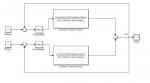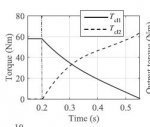# Using a PID controller in simulink to simulate shift jerk in dual clutch transmission

#### HastiHello. I need to simulate a dual clutch transmission shift process in matlab for my university project. I want to use a PID controller to minimize shift jerk. I have my transfer functions .My input is time derivative of clutch torque.
There are two clutches. I know the start and end point of each clutch torque during shift process.
My goal is that i connect this points so that the jerk becomes minimum. My problem is i do not have the input value not at the beginning nor the end.
How should i tell matlab to choose the inputs(derivative of torque) while the torque at the start for example is zero and its final value is 60.
i attach the torque-time diagram that i should get to and my controller that i designed.
Thank you

#### ControlsGuy25

View attachment 601View attachment 602
Hello. I need to simulate a dual clutch transmission shift process in matlab for my university project. I want to use a PID controller to minimize shift jerk. I have my transfer functions .My input is time derivative of clutch torque.
There are two clutches. I know the start and end point of each clutch torque during shift process.
My goal is that i connect this points so that the jerk becomes minimum. My problem is i do not have the input value not at the beginning nor the end.
How should i tell matlab to choose the inputs(derivative of torque) while the torque at the start for example is zero and its final value is 60.
i attach the torque-time diagram that i should get to and my controller that i designed.
Thank you
Hello !

Try this excellent article it using triple step non linear, with feedforward cascade control !